# Crystal Structures Assignment Help

## Crystal Structures

Crystal structure is defined as the arrangement of atoms, ions, or molecules in a crystal. Crystals are solids having, in all three dimensions of space, a regular repeating internal unit of structure.

In other words - A crystal is a periodic array of atoms. In mineralogy and crystallography, crystal structure is a unique arrangement of atoms or molecules in a crystalline liquid or solid. A crystal structure is composed of a pattern, a set of atoms arranged in a particular way, and a lattice exhibiting long-range order and symmetry. Many elements and quite a few compounds are crystalline at low enough temperatures, and many of the solid materials in our everyday life (like wood, plastics and glasses) are not crystalline.### Rocksalt [Sodium Chloride (NaCl)] Structure:

It is typically formed by the evaporation of salty water (such as sea water) which contains dissolved Na+ and Cl- ions. The structure of Rocksalt has high ionic bondings. In this structure large anions are arranged closely contained by a cube. All the octahedral interstitial positions are filled with cations in this structure.

You can easily understand the crystal structure of rock salt (NaCl) as follows --

(i) rNa+ = 96 pm rCl– = 181 pm [pm = picometer]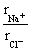=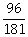= 0.524 this ratio lies between 0.414 and 0.732 and coordination number is thus 6.

(ii) Cl– forms FCC type packing

(iii) Na+ ions occupy all octahedral voids

(iv) Coordination number for Na+ = 6

Coordination number for Cl– = 6

(v) Number of Na+ ions per unit lattice = 4

Number of Cl– ions per unit lattice = 4

Hence total number of ions = 8

Hence for each Na+ ions there is only one Cl– ions.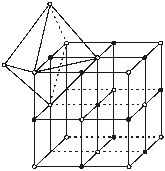(A unit lattice consists of 4 formula units of NaCl or vice versa if NaÅ forms unit lattice).

Other examples of this type are halides of Li, Na, K, Rb, fluoride of Cs oxides and sulphides of Mg, Ca, Sr, Ba, AgCl, AlBr, AgF.

### Cesium Chloride (CsCl) Structure

Caesium chloride is the chemical compound with the formula CsCl. CsCl is a colorless solid inorganic compound and important source of caesium ions. Cesium chloride used in a variety of applications like centrifugation and cancer therapy.

(i) rCs+ = 169 rCl– = 181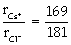= 0.93 Hence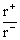lies between 0.732 to 1.0

Thus coordination number is = 8 (As we know CsCl has BCC type structure)

(ii) Cl– form BCC packing (iii) Cs+ ions occupy the body centred void of the lattice

(iv) Each Cs+ is surrounded by 8 Cl– ion

Each Cl– is surrounded by 8 Cs+

(v) Number of Cl– ions per unit lattice = 1

Number of Cs+ ions per unit lattice = 1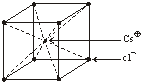Other examples of this type of chemical compound are CsBr, CsI, NH4 Br, NH4I, Thallous cyanide and Thallous chloride.

### Zinc Blende [(Zn,Fe)S] Structure

Zinc blende, also known as sphalerite, is an important ore from which zinc metal is obtained. It consists largely of zinc sulfide in crystalline form but almost always contains variable iron.

(i)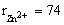pm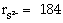pm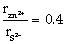This value lies between 0.225 and 0.414 hence a tetrahedral void is occupies coordination no. = 4

(ii) S2– ions are arranged in FCC types structure

(iii) Zn2+ ions occupy half the tetrahedral void.

(iv) Number of Zn2+ ions = 4

Number of S2– ions = 4

Theshould have been 0.225 but the value is 0.40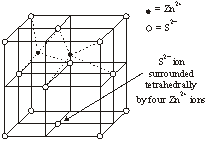The close packed arrangement of S2– ion opens up and accumulates the larger S2– ions

(v) Coordination number of Zn2+ = 4

Coordination number of S2– = 4

(vi) The formula unit for ZnS type lattice = 4

Other examples are : AgI, Beryllium oxides have Zinc blende structure.

### Calcium Fluoride (CaF2) Structure

Fluorite (also called fluorspar) is a halide mineral composed of calcium fluoride. It is an isometric mineral with a cubic habit.Fluorite is one of the most colorful minerals on earth. The range of common colors for fluorite are purple, blue, green, yellow, colorless, brown, pink, black and reddish orange. It is easy to see why fluorite earns the reputation as "The most colorful mineral in the world".

(i) Ca2+ is arranged in FCC type structure

(ii) F– occupy all tetrahedral voids

(iii) Each Ca2+ surrounded by 8 F– ion

Each F– is surrounded by 4 Ca2+ ion. Thus gives a (8:4) coordination number. CaF2 type unit cells are present in SrF2 and CdF2.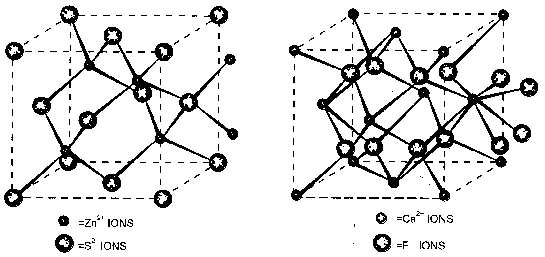### Antifluorite (Na2O) Structure

(i) O2– form F.C.C

(ii) Na+ occupies tetrahedral voids

(iii) Coordination number of O2– = 8

(iv) Coordination number of Na+ = 4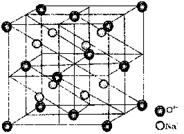### Corundum (Al2O3) Structure

(i) O– – ions form hcp

(ii) Al3+ occupes two third octahedral voids

(iii) Other examples are Fe2O3, Cr2O3, Mn2O3 etc.

### Diamond (Corbon) Structure

The crystal structure of a diamond is a face-centered cubic or FCC lattice. Each carbon atom joins four other carbon atoms in regular tetrahedrons (triangular prisms). Based on the cubic form and its highly symmetrical arrangement of atoms. Diamond is the ultimate gemstone, having few weaknesses and many strengths. It is well known that diamond is the hardest substance found in nature.

(i) ‘C’ form fcc unit cell.

(ii) ‘C’ atoms are also present at alternate tetrahedral voids.

(iii) C.N = 4.

(iv) Total ‘C’ atoms per unit cell is 8.

### Customer Support Services (live 24/7)

We are ready to help you on many chemistry chapter like Crystal structures, Structure of the atoms(nucleus, proton, neutron and electron) and Chemical bonding. If you seek Assignment/Homework help in chemistry, our expert online chemistry tutors are available around the clock (24/7) to help you in crystal structures, chemical reactions and chemical bonding.

We are the leading online Assignment Help provider in chemistry and physics. Find quality answers to all of your doubts regarding the crystal structures in chemistry. We at assignmenthelp.net provide homework/Assignment Help to the school, college or university level students. Our Chemistry Assignment Help service is focused on: on time delivery, excellent quality, creativity, originality and free plagiarism.